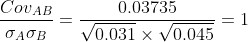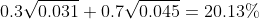Scan QR code or get instant email to install app

Question:

# A portfolio is invested 30% in Asset A with the remainder invested in Asset B. Asset A has an expected return of 6% and variance of returns of 0.031, while Asset B has an expected return of 7% and variance of returns of 0.045. The covariance between the returns of the two assets is 0.03735. The standard deviation of returns for the portfolio is closest to:

A 20%.
explanation

1212The correlation between the returns of the two assets is:Therefore, the standard deviation of the portfolio returns is a weighted average of the standard deviations of returns for the two assets:.
Since the correlation of returns is +1, there are no diversification benefits.